# Is Optimizing your Neural Network a Dark Art ?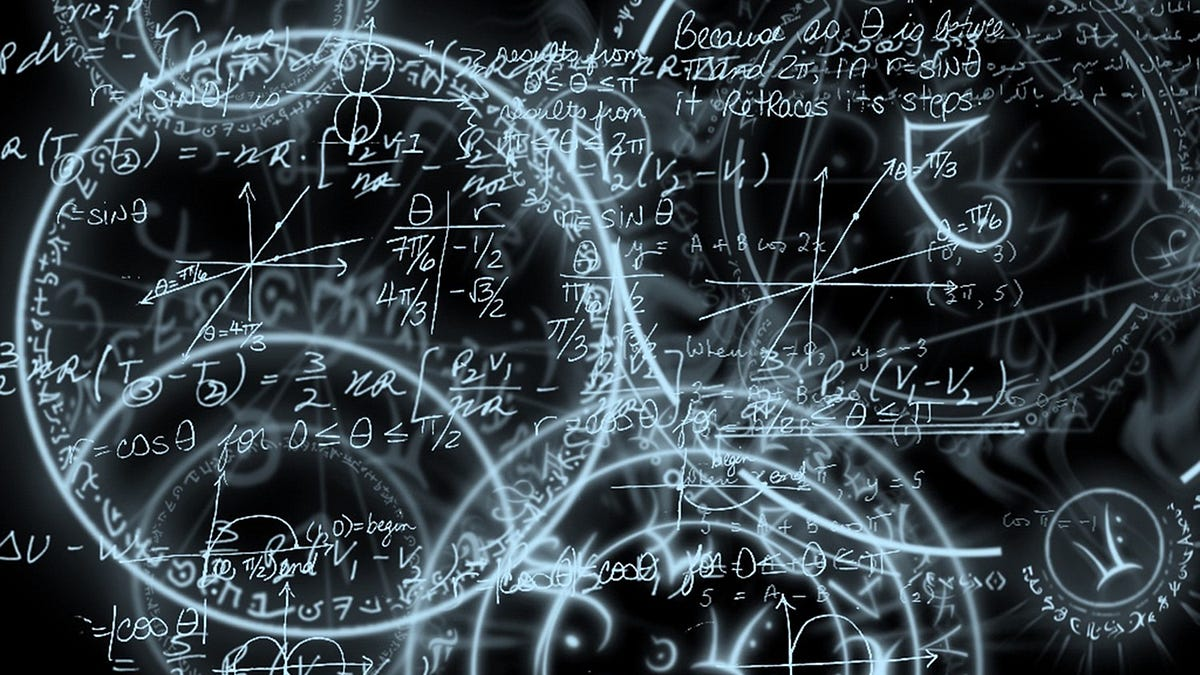photo credit

Just like in real life, different people learn at different pace, using different techniques and understand or retain qualitatively different aspects of what they have learnt. Artificial Neural Networks or ANNs are no different. ANNs share the same pitfalls of the brain, while it tries to replicate the strengths of the brain. In other words, they learn at different pace, using different techniques, and retain qualitatively different aspects of information.

It is almost considered a dark-art to optimize very large scale AI and ANN systems, and in this post, I want to touch upon the high-level aspects of possible issues that can occur during learning and provide a summary-explanation on the existing techniques to counter them.

If you need a quick introduction to ANNs and its workings, I have added the links to the previous posts as a reference:

Learning is a procedure where we are searching for the best-fit value for the knowledge weights of the ANN in order to minimize the global error. To do so, we found a mechanism to update the weights of the ANN using error derivates and gradient descent as follows: (note that this is a basic equation)

We established that the rate of change of error with respect to the hidden knowledge weights, is a function of the output of the activities of the network.

This is not as straight forward as it seems. There are several other optimization decisions that needs to be made before the learning can begin and/or is optimal. Questions such as:

• What type of ANN to choose?
• What should be the size of the ANN architecture? In other words, how many input neurons, hidden neurons, hidden layers and output neurons are needed?
• Is there a way to initialize the network so that number of iterations in training can be reduced?
• Is there a way to bias the network?
• What is the best way to use the error derivatives?
• How to avoid overfitting?

Let’s break down each of this and see what techniques are available.

#### Types of ANN

While there are innumerable types of ANN, they can be broadly classified into the following:

Feed Forward Neural Networks (FFNN)

This is the simplest type of ANN. Information moves in a single direction, from input layer to hidden layer to output layer. Typically they are single layered (not always) and good enough to encapsulate behavior to classify textual data. Activation functions are sigmoidal in nature and uses simple backpropagation for learning. You can use FFNN extremely well in large scale classification systems with noisy data.

Convolutional Neural Networks (CNN)

CNNs are a type of FFNN where information flows in single direction. CNNs are modeled after the visual cortex in animals. CNNs are multi-layered. Each layer of CNN has portions of neurons which process a focused portions of the image (which can overlap). They use techniques like tilting the image for further layers to process. This creates many different copies of the same image in different positions, which increases visual accuracy. This technique is also called replicated features apporach. CNNs are heavily used in computer vision and visual pattern recognition domains.

Recurrent Neural Networks (RNN)

Unlike FFNN, a RNN is bi-directional. Which means, information can flow back into neurons after activations. RNNs are memory based models (similar to any Linear Dynamical Models or Hidden Markov Models). They are quite powerful as they not only allow memory of past states, but also allow non-linear, dynamic, temporal behavior that can update the states. While RNNs are powerful, they are quite unstable relative to simple FFNNs. RNNs are used in applications where speech translations, signal processing, motor control, Natural Language Interface (NLI/NLP), or text prediction is needed.

There are inumerable variations of the above types of ANNs to custom fit specific applications. Also, the nature of activation functions used, transfer potential functions used, training and cost functions used produce different types of networks.

#### How to determine the size of the ANN?

The size of the network is a function of the input and output expected. There are three main factors of input and output of an ANN.

1. The dimension of the input vector.
2. The number of samples in the input training set.
3. The number of classes in which the inputs needs to be classified as output.

If you want to train the ANN to recognize the picture of a cat, you have to determine the size of each cat picture (which should be a constant), and the total number of pictures used for training.

The size of the cat picture can be defined as the x and y pixel co-ordinates of the cat. Let’s say we use a cat picture of size 200 * 200. Then we have 40K pixels that we are working with. 40k shall be the total number of input neurons needed that shall represent the state of every pixel. (The state is always represented as a real-valued number which can be the RGB value of the pixel)

The number of samples in the training set can be 500 cat pictures.

Now, we need to determine what is the total number of classifications the cat should be categorized into. Do we just want a true/false output to state if the input picture is a cat or not? Or, do we want the output to be a specific breed of cat to distinguish between a Siamese cat and a Abyssinian cat?

Let’s say, I want a single output of true/false (0 or 1) for now. Hence the total number of output neuron shall be 1.

Now that we have the input size and the output size, we can use a thumb rule as follows to determine the size of a simple FFNN.

• Let, N(i) be total number of input neurons.
• N(s) be the sample size.
• N(o) be the total number of output neurons.
• We need to find N(h), the total number of hidden neurons needed.
• Let, ‘alpha’ be some scaling factor between 2 and 10.

We have the following equation: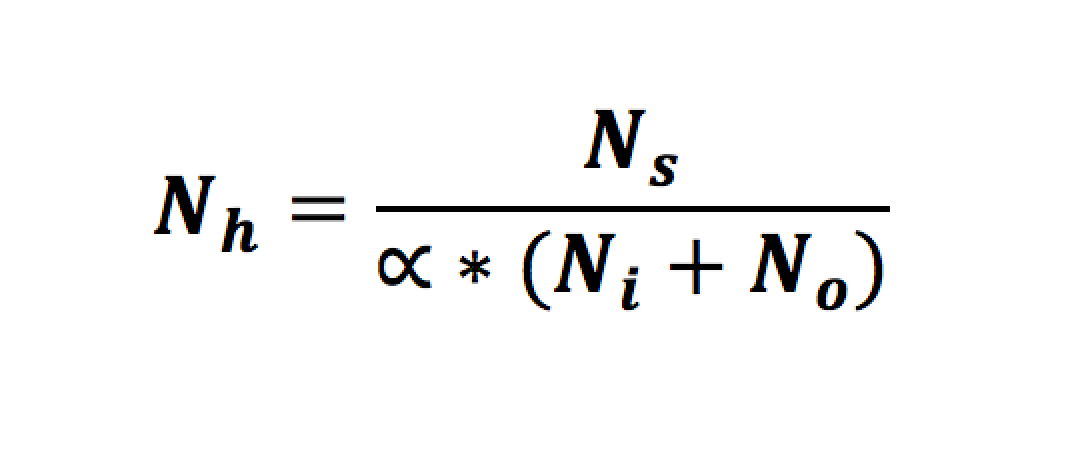Thumb rule to determine the number of hidden units needed

You can use the scaling factor for tuning the network based on the complexity of the input domain.

• Smaller networks consume less resources (number of free parameters to update is minimal) and take minimal compute cycles and storage space. But they are not necessarily accurate (gets stuck in local minima).
• Larger networks are resource consuming (compute cycles, storage space) but can encapsulate better behavior and accuracy and are more fault tolerant.

There is no magic number to figure out the number of hidden units needed. Also note that the hidden units can be laid out in multiple layers (The input layer and output layer shall always be a single layer).

To choose the optimal size of the network, you can use couple of different approaches.

1. Cascading up (called cascade correlation) from a smaller network: In this approach, you choose the minimal number of hidden units and verify if the accuracy of the network is good enough. If not, you increase the size to the next minimal possible level and verify the efficiency and accuracy again. In effect you are moving up until you are satisfied with the results.
2. Pruning down from a larger network: Here, you start with a possibly large network and prune out the dead spaces of the network and adjust the remaining weights of the network to accommodate the removal of the hidden units. This is typically a post-training method, using approaches like conjugate gradients and is a bit more complex than cascade correlation.

Complex behaviors need very large networks nonetheless. Today, mid-2016, there is a practical hardware limit in how large a network can get. Google is meant to have systems with several billion connections.

Trivia: Did you know the number of connections in a human brain can run to 100 trillion connections, connecting 100 billion neurons in such a compact space? That is 1000 times the number of stars in our galaxy. And, it runs on relatively lower energy compared to the artificial hardware behemoths by consuming somewhere between 300 to 1500 calories every day. A true marvel we humans are. The AI systems is quite far from getting such uber-powered hardware anytime soon.

#### How to initialize your network?

We understood that training is a process that adjusts the weight of the connections in hidden neurons to a number optimal to exhibit behaviors that is expected of it.

Can all weights be zero to start with? How do these weights start? If all weights starts with zero, then the transfer potential shall always be zero (from the following equation) and shall be useless.

What if everything start with one? In this case, if hidden units have exactly the same incoming and outgoing weights, then they shall land up getting exactly the same gradient. Then, all units will have exactly the same gradient. Which is quite useless as they can never learn different features of the input.

Then should we just start assigning a +1 to every connection starting from the first connection? as in, the first connection gets a weight 1, the second connection gets a weight 2, the third a weight 3 etc..? This also has potential problems. Note that every unit has several incoming connections and hence, the transfer potential can quickly become a very large number. Even small changes to the weight gradients in this case will over-shoot learning.

So, what did we learn? We said that, the weights cannot be the same number nor symmetrical. Also the weights should not be a large number.

So what should the weight initialization be? The weights should be a non-zero, random (non-symmetrical), small fraction (positive or negative) to get the network started. Typically, all ANNs are initialized this way.

It is observed that if 50% of the network contains a negative weight and 50% positive weight randomly with non zero real-value in range -1 to 1, then its a good place to start. If you take a weight histogram of 1000 random real-valued weights in range -1 to 1, you get to see a plot similar to the following:

While, this is how the network starts with its weight, is this how the network ends up after full training? is there a pattern in how these number form after learning? do they shape-up in a particular way specific to the input? do they cluster in a particular manner?

Over innumerable number of observations, it is found that, in-general, a fully trained Neural Net does NOT have their weights distributed equally (as shown in the start of the network). Instead, it is observed that a large number of weight is tightly clustered around the zero-mean while the rest of the numbers are spread out (This pattern can deem different based on the transfer potential and activation functions used. This pattern is true for most sigmoidal activations though).

The following illustration shows the histogram from a gaussian function which shows that by controlling the standard deviation, we can cluster the weights more closely to zero-mean during initializtion.

So, instead of spreading the weight range, randomly-equally, between the range -1 to 1, there are techniques to initialize them in a way that the network starts with a tight clustering around zero-mean. The reason to do this, is to improve the efficiency of the network to identify different features of the network from get-go, which helps in better training.

Here are some sample weight initialization techniques: (To retain the sanity of reading-time of this post, I have purposefully kept the hints to high level. Will explain the techniques and merits in detail in future posts..)

• Initialize weights to be proportional to squareroot of the number of fan-ins.
• Use a gaussian noise while initializing the random weights.
• Use a Nguyen-Widrow initialization mechanism.
• If using ReLU as activation, use Xavier weight initialization techniques.

#### Is there a way to bias the Neural Network?

For sigmoidal activation functions, we noticed that the curve thresholds at zero-mean and starts moving from a output value of 0.5 towards 1, as shown in following illustration (assuming that the weight is 1) :

A better way to interpret the above illustration, is to understand that the activation function outputs a value 0.5 when the input value x is zero.

What if we wanted to bias the network in such a way that the activation function outputs 0.5 when the value of x is 5? In other words, is there a way to bias the network to threshold up for input value 5?

To do so, you have to introduce a bias term to the “transfer potential” as follows: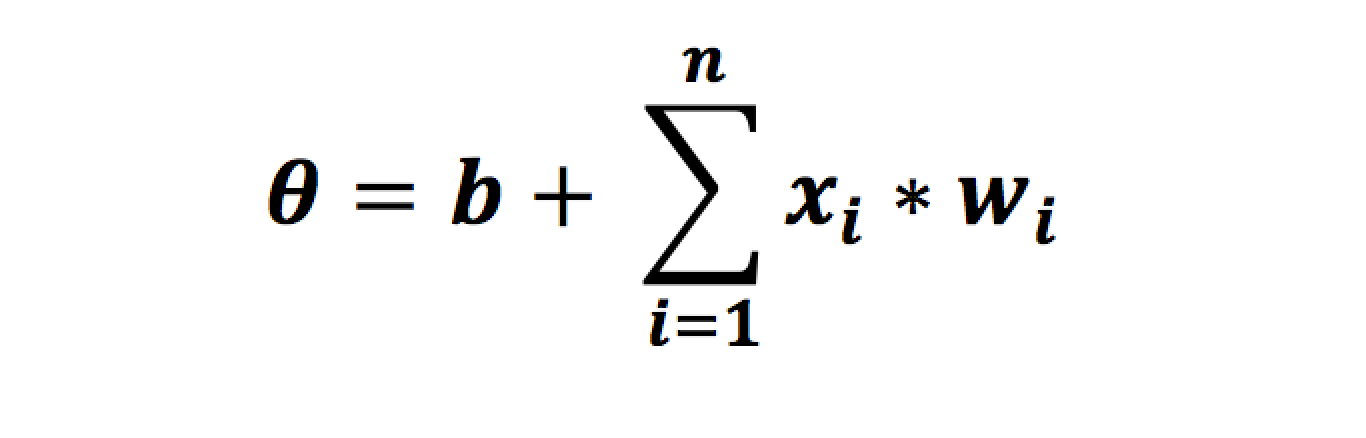transfer potential with bias neuron

The parameter ‘b’ stands for bias. So if we need the network to threshold at around input value of 5, then we can introduce a bias value of -5 (Since this is sigmoidal, its intuitive)

In other words, the network would like as shown: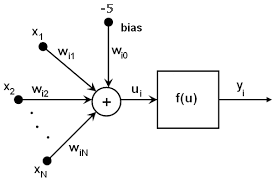Network with a bias of negative 5

Now the output of the sigmoidal activation function would look as illustrated:

Notice that with a negative bias, we have effectively shifted the sigmoid to the right. Similarly, if you want to shift the sigmoid to the left, then you can introduce a positive bias as follows:

In the above illustration, notice how the curve switches over and thresholds for x = -5.

In effect you are biasing the activities of the neurons to behave in a particular manner. Here is a collective illustration of all curves plotted on the same graph to emphasize the bias.

#### How often to update the weights?

Since training a ANN is a resource intensive activity, one of the questions that comes to mind is, do we have to update the weights everytime for a single pass of the input? If we have a 1000 pictures of cat:

• Online weight updates: We update the weights for every single pass of the input. Here a single pass is considered as 1 iteration. If we have 1000 pictures of cat to train, then we update 1000 times. This is quite resource intensive.
• Epoch (or full-batch) weight updates: We update the weight only once after aggregating the errors for every pass. Here we have a single iteration for all the 1000 pictures. This is quick, but does not learn the errors well.
• Batch (or mini-batch) weight updates. We break down the input into smaller batch sizes [a log(n) of the number of inputs, where n is between 2 and 10]. So, we can have 100 pictures per batch which gives 10 iterations for a input size of 1000. This uses less resource and you can backprop the gradient more often to train the network more effectively.

#### How much of the weight to update?

Another question that plagues the optimization is how much of the error derivative should we use to update the weights? This is a important question. If we keep applying the full derivative to the weights as shown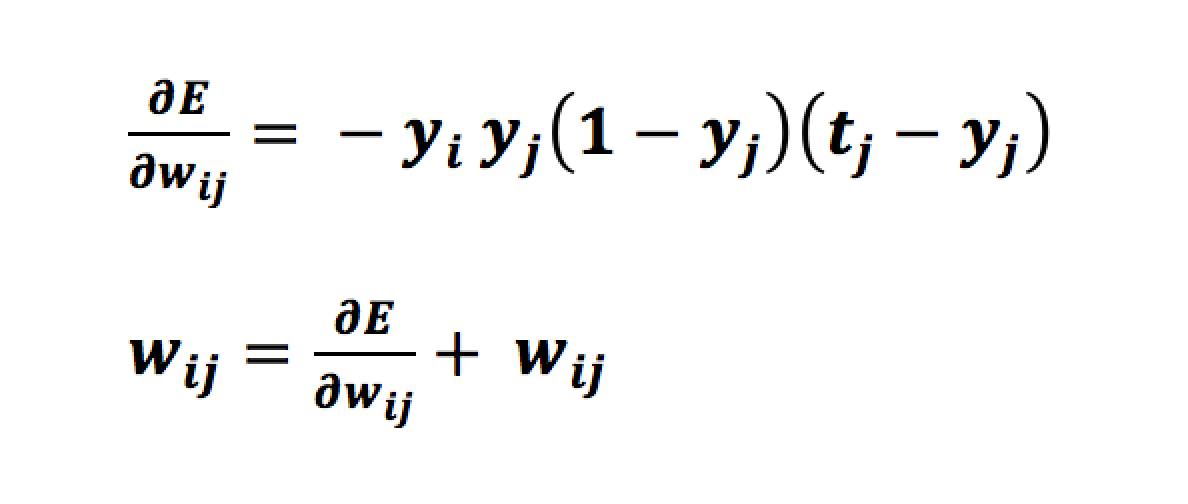Weight update with the full derivative

then, the full error derivates applied as-is might be introducing large step-jumps. The network may not converge quickly and the error may randomly oscillate and in worst cases many not converge at all, as illustrated:

Instead, we can control the scale of learning and the velocity of change.

There are two specific parameters that are used to do so:

• An ‘epsilon’ parameter that controls the learning rate. This parameter slows down or speeds up learning by controlling how much proprotion of the error derivative should be applied.
• An ‘alpha’ parameter that controls the momentum value. This parameter controls the percent of previous weight-derivate [previous delta, denoted by ‘delta’ w(t-1)] to be used during current weight updates. This value is helpful in training the network out of local minima.

The new updated equation looks as follows:

There are other techniques for better backpropagation as well as follows:

• Resilient Propagation (or RPROP)
• RMS Propagation
• LMA Training

(More on these techniques later)

#### Overfitting

ANNs are very powerful models to train high-dimensional data. ANN performs well over other statistical machine learning models because of its ability to work with high-dimensional, noisy data.

Due to this, there are some pitfalls to these models that trains on high-dimensional data. one of the major pitfall is called overfitting.

To understand overfitting, in the case of the 1000 cats picture being used for training, if most of the cats were brown in color and only had a front profile of the cat, then the system pretty much learns to only recognize front-profile-of-brown-cats as cats and may provide low confidence values for other cat pictures. The reason this happens is because, the model is almost “memorizing” the pictures as against “learning” the features of the cat. Now if you add-in ambient noise into the pictures like trees in the background or window sills, this gets to memorize only cats on trees as cats etc..

To reduce overfitting, its important to regularize the learning in such a way that the model can in-general recognize all cat pictures. This is also called generalization. The following are different techniques to regularize the learning

• Have large quantities of Data. Also, have a wider variety of data for training.
• Use Cross-validation technique where you set aside a portion of the training data to train and another portion as a validation set for testing.
• Use a optimal network size. Large networks with too many free parameters, lands up memorizing the features. Reduce the size of the network in this case.
• Early stop while training the model where you don’t allow the model to over-train and memorize too many details of the inputs.
• Apply weight decay for large weights in order to restrict the training to overshoot.
• Add bias and noise into the system (during weight updates, or in transfer potentials, or in activation functions)
• Create copies of the same ANN at different stages of training (early stop at different stages) and average the output of the replicas.
• Have different size of the same type of ANN and average the outputs.
• Have different type of ANN all together and average the outputs.
• Use Drop-out, as in, randomly drop connections of the network during training. This simulates averaging of multiple network within the same model.

To conclude, we learnt about different issues during optimizing an ANN and some techniques to avoid the issues. While these are only indicative issues and base techniques, the entire work of getting results from your ANN and AI systems relies in your ability to tune every aspect of the system to work optimally. There are tons of techniques to do so.

In effect, tuning such complex systems is part science, part art, part black-magic and part luck and need a whole lot of patience (and resource) for large systems. Happy Tuning.